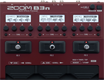# Factory : Funky SynB

Discussion in 'Zoom B3n' started by Tonelib, May 20, 2018.

1. Factory : Funky SynBDevice: Zoom B3n
Firmware: 2.00

Name on device: Funky SynB
Optimized for: Phones/Speaker

Effects chain:This tight synth bass sound uses Z-Syn and has quick response and good note separation.

Effect: "Z-Syn" (Sfx), active - "yes"
"Frequency" = 6
"Range" = 8
"Decay" = 58
"Resonance" = 13
"Wave" = SAW
"Tone" = 8
"Balance" = 40
"Volume" = 56
"Frequency" = 2
"Range" = 8
"Decay" = 72
"Resonance" = 18
"Wave" = SAW
"Tone" = 7
"Balance" = 50
"Volume" = 43
"Parameter set" = A

Effect: "Ba Octave" (Modulation), active - "yes"
"Oct" = 9
"Lo" = 0
"Hi" = 9
"Dry" = 100

Effect: "AMPG SVT" (Amp simulator), active - "yes"
"Bass" = -2.0
"MID-F" = 300Hz
"Middle" = 0.0
"Treble" = 2.0
"Gain" = 25
"Ultra" = OFF
"Volume" = 63
"SOLO" = 3

Patch Volume: 100